• 1
•
• 2
•
• 2
•
•
•
5
Shares

# Velocity Selector or Wien filter

## Introduction

We know that when a charged particle travels through the cross-field (perpendicular electric and magnetic field) then each field exerts a force on the traveling charged particle and tried to bend its trajectory.

If I asked you, at what speed should a charged particle traveled in the cross-field so that it experiences zero net force and altogether there no change in its path also.

So this present article, we will discuss the velocity at which particle experiences zero net force. In fact, we will discuss velocity selector or Wien filter.

## What is a velocity selector?

Velocity selector or Wien filter is a device consist of a perpendicular electric and magnetic field that can be used to select the charged particle of specific velocity which when injected into the cross-field then it experiences zero net force.

This device is composed of perpendicular electric and magnetic fields. When the charged particles are injected into this field then it is observed carefully that which particle is deflecting and which particle is not.

The particle which is not deflected by the cross-field traveled in a straight line. Non-deflecting particle is selected as special particle having special velocity given as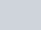.

It is used in accelerator mass spectrometry to select the charged particles based on their velocity. This device is named after the physicist Wilhelm Wien who developed it in 1898 for the study of anode rays. It can be composed as a charged particle energy analyzer, monochromator, or mass spectrometer.

## The concept behind the velocity selectorfig. 2, use of velocity selector in the mass spectrometer, source: http://hyperphysics.phy-astr.gsu.edu/

If a charged particle is moving in perpendicular electric and magnetic fields then we know that a charged particle moving in an electric field experiences an electric force proportional to the charge (q) and field strength (E). The electric force experienced by the charged particle is given as-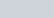Where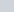is the force by the electric field, q is the charged particle and E is the strength of the electric field.

Similarly, a charged particle moving in a magnetic field also experiences a magnetic force proportional to the velocity (v), charged particle (q), and strength of the magnetic field (B). The magnetic force experienced by the magnetic field is given as-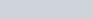Where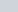is the force by the magnetic field, q is the magnitude of the charged particle, B is the strength of the magnetic field, and cross (×) is the vector cross product between velocity (v) and magnetic field (B).

In the case of a velocity selector, the velocity of the charged particle is always perpendicular (90°) to the magnetic field. So the magnetic force exerted on the charged particle becomes-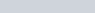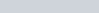The direction of the magnetic force is specified by the vector cross product and it is always perpendicular to the velocity and the magnetic field.

Setting up the electric and magnetic force in equal magnitude and opposite directions, then both equations of forces (electric and magnetic force) will be equal and can cancel each other so that the charged particle experiences zero net force. Equating both forces, we get-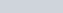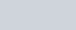We see that the velocity of the charged particle in the Wien filter is independent of the magnitude of the charge and mass of the charged particle. The charged particle whose velocity is equal to the ratio of electric field and magnetic field can travel through the crossed field without any deflection in a straight line.

## Uses of velocity selector

Velocity selector can be used in-

## Limitations of velocity selector

Some limitations of velocity selector are given below:

• The velocity of the charged particle in the velocity selector is independent of the mass and charge of the particle means, particles of any size and charge can pass the filter.
• The uncharged particles can also travel through the velocity selector.

Stay tuned with Laws Of Nature for more useful and interesting content.

0%
4Test your knowledge on velocity selector or wien filter

Choose the correct option given below the question and check your score and answers at the end of the quiz.

1 / 5

1. Velocity selector is used in

2 / 5

2. Wien filter is developed by

3 / 5

3. In a velocity selector, if a charged particle passes the cross field without any deflection then the velocity of the charged particle is given by

4 / 5

4. In the case of velocity selector, the velocity of the charged particle is perpendicular to the

5 / 5

5. Velocity selector is also known as

The average score is 90%

0%